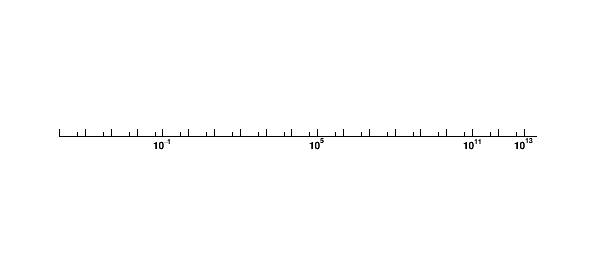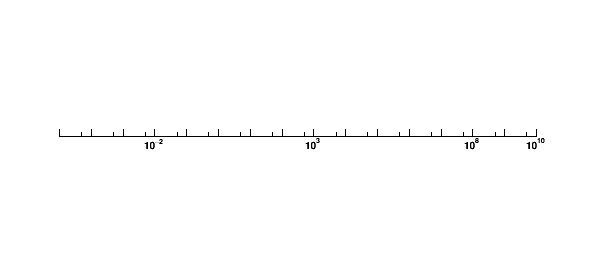# Axis labels set in log scale

Dear experts

I want to draw an axis ranging from 1e-5 to 1e13 in log scale and set the label location at the 1e-5, 1, 1e5, 1e10. My code and result as follows:

``````void test(){
TCanvas* c1 = new TCanvas("c1","",10,10,600,300);
c1->Range(-10,-1,10,1);

TGaxis *a1 = new TGaxis(-8,0,8,0,1e-5,3e13,3,"G");
a1->SetName("a1");
a1->Draw();
}
``````I changed the axis range to [1e-5, 1e10]. But it failed as well.How could I modify my code to obtain the expected result?

``````   TGaxis *a1 = new TGaxis(-8,0,8,0,1e-5,3e13,510,"G");
``````

Thanks, couet.

I found reference on https://root.cern.ch/doc/master/classTGaxis.html and explanation for the parameter ndivision. But how this factor works when I use the log scale? I have noted the effect when I choose linear axis. Is there any way to divide the axis just three division, at 1e-5, 1, 1e5 and 1e10?

The number of divisions is managed a bit differently in case of log. It is more the number of labels drawn on the decades.They are distributed along the axis but all the decades are drawn. The exact labelling you are asking is not possible just playing with the number of divisions. The only way I can think of would be to draw the axis showing the labels you need and then remove the labels you do not want. The labels you need are shown when the number of divisions is at least equal to 15. The following macro does what you want:

``````{
TCanvas* c1 = new TCanvas("c1","",10,10,600,300);
c1->Range(-10,-1,10,1);

TGaxis *a1 = new TGaxis(-8,0,8,0,1e-5,3e13,15,"G");
a1->ChangeLabel(2,-1,0.);
a1->ChangeLabel(3,-1,0.);
a1->ChangeLabel(4,-1,0.);
a1->ChangeLabel(5,-1,0.);
a1->ChangeLabel(7,-1,0.);
a1->ChangeLabel(8,-1,0.);
a1->ChangeLabel(9,-1,0.);
a1->ChangeLabel(10,-1,0.);
a1->ChangeLabel(12,-1,0.);
a1->ChangeLabel(13,-1,0.);
a1->ChangeLabel(14,-1,0.);
a1->ChangeLabel(15,-1,0.);
a1->ChangeLabel(17,-1,0.);
a1->ChangeLabel(18,-1,0.);
a1->ChangeLabel(19,-1,0.);
a1->Draw();
}
``````

Thank you very much, couet.

A bit more compact.

``````{
TCanvas* c1 = new TCanvas("c1","",10,10,600,300);
c1->Range(-10,-1,10,1);

TGaxis *a1 = new TGaxis(-8,0,8,0,1e-5,3e13,15,"G");
for (int i=2; i< 20;i++) {
if (i==6 || i==11 || i==16) continue;
a1->ChangeLabel(i, -1, 0.);
}
a1->Draw();
}
``````
1 Like

This topic was automatically closed 14 days after the last reply. New replies are no longer allowed.